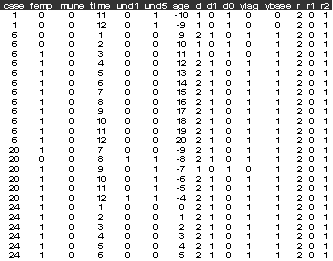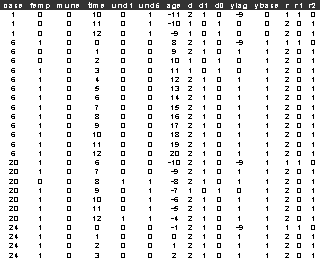Exercise FOL3 Binary Response, Female Labour Force Participation in the UK

Davies, Elias and Penn (1992) and Davies (1993) as part of the ESRC funded Social Change and Economic Life Initiative. The data we use is the annual employment behaviour of wives from Rochdale (UK) from the date of their marriage to the end of the survey in 1987. The binary response femp takes the value 1 if a wife was employed in the current year and 0 otherwise. There is a set of explanatory variables that include husband's employment status and age (years). In this exercise we are going to see if we can distinguish state dependence (first order effects) in employment behaviour of wives from unobserved heterogeneity. The same data (wemp.dta) were used by Rabe-Hesketh and Skrondal (2005, exercise 4.5).

References

Davies, R.B., Elias, P., and Penn, R., (1992), The relationship between a husband's unemployment and his wife's participation in the labour force, Oxford Bulletin of Economics and Statistics, 54, 145-171

Davies, R.B., (1993), Statistical modelling for survey analysis, Journal of the Market Research Society, 35, 235-247.

Rabe-Hesketh, S., & Skrondal, A., (2005), Multilevel and Longitudinal Modelling using Stata, Stata Press, Stata Corp, College Station, Texas

Conditional analysis

Data description (wemp_base2.dat)

Number of observations = 1274

Number of cases = 144

The variables include the following:

case= identifier for wives

femp=1 if wife is in employment status in current year, 0 otherwise

mune=1 if the husband is in employment in current year, 0 otherwise

time=year of observation-1975

und1=1 if the wife has children under the age of 1, 0 otherwise

und5=1 if the wife has children under the age of 5, 0 otherwise

age=wife's age-1975

d=1 if mmmmmm, 2 otherwise?

d1=1 if d=1, 0 otherwise

d0=1 if d=2, 0 otherwise

ylag=femp lagged 1 year

ybase=femp in 1st year

r=2 for all post 1st year observations

r1=0 for all observations

r2=1, if r=2

The first few lines of wemp_base2.dat look like:Exercise

1)      Estimate a heterogeneous logit (level-2 with case, mass 20) model of female employment participation (femp), with a constant and the lagged female employment participation variable (ylag), mune, und5, and age regressors.

2)      Add the initial condition of employed in the 1st year (ybase) to the previous model. How do the inference on the lagged responses (ylag) and the scale effects differ between the two models.

Joint analysis of the initial condition and subsequent responses

Data description (wemp_base.dat)

Number of observations = 1425

Number of cases = 151

The variables are the same as wemp_base2.dat except that this time the variables ylag, r, r1 and r2 take more values.

ylag=femp lagged 1 year, -9 if its the 1st year

r=1, for the initial response, 2 if a subsequent response

r1=1 if d=1, 0 otherwise

r2=1 if d=2, 0 otherwise

The first few rows of wemp_base.dat look likeExercise

1)      Estimate a common random effect common scale joint logit model (mass 20) of female employment participation (femp). Use constants in both linear predictors. Use the d1 and d2 dummy variables to set up the linear predictors. For the initial response use the regressors: mune, und5, and age regressors. For the subsequent responses use the regressors: the lagged female employment participation variable (ylag), mune, und5, and age. What does this model suggest about state dependence and unobserved heterogeneity?

2)      Re-estimate the model using a bivariate model for the random effects (common scale). What is the value of rho? Is this a significant improvement over the common scale parameter model?

3)      To the bivariate model add the initial or baseline response (ybase). Does this make a significant improvement to the model?

4)      Compare the results obtained for the various models on the covariates and role of employment status in the previous year. Are both state dependence and unobserved heterogeneity present in this data? Do the results on the covariates make intuitive sense?